Mathematical and Physical Journal
for High Schools
Issued by the MATFUND Foundation
 Already signed up? New to KöMaL?

#Problem A. 704. (October 2017)

A. 704. A regular triangle has side length $\displaystyle n$. We divided its sides into $\displaystyle n$ equal parts and drew a line segment parallel with each side through the dividing points. A lattice of $\displaystyle 1+2+\cdots+(n+1)$ intersection points is thus formed. For which positive integers $\displaystyle n$ can this lattice be partitioned into triplets of points which are the vertices of a regular triangle of side length $\displaystyle 1$?

Proposed by Alexander Gunning, Cambridge, UK

(5 pont)

Deadline expired on November 10, 2017.

Answer. There exists a construction exactly when $\displaystyle n\equiv -1,1,8,10\pmod{12}$.

Solution (by the proposer). There exist obvious constructions for $\displaystyle n=-1$ (i.e., an empty grid of points; we may use this base case in our induction) and $\displaystyle n=1$. Moreover, as seen in the diagram below,

$\displaystyle \bullet$ for $\displaystyle n\equiv -1\pmod{3}$, one can extend the construction to $\displaystyle n+2$,

$\displaystyle \bullet$ for $\displaystyle n\equiv 0\pmod{2}$, one can extend the construction to $\displaystyle n+3$,

$\displaystyle \bullet$ for $\displaystyle n\equiv 1\pmod{2}$, one can extend the construction to $\displaystyle n+9$.

Therefore, by induction, there exists a construction for $\displaystyle n=12k-1$, $\displaystyle n=12k+1$, $\displaystyle n=12k+8$, and $\displaystyle n=12k+10$ for any $\displaystyle k\ge 0$.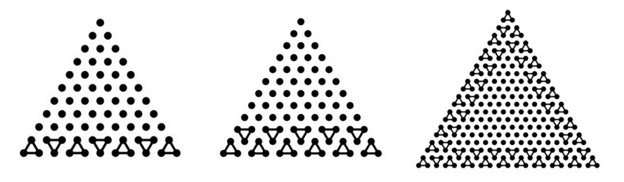To prove that there is no construction for any other $\displaystyle n$, first note that $\displaystyle 3$ divides $\displaystyle 1+2+\dots+(n+1)=\frac{(n+1)(n+2)}{2}$. Then the lattice can be colored using the three colors red, blue, green such that points of distance $\displaystyle 1$ have different colors and each color is used $\displaystyle \frac{(n+1)(n+2)}{6}$ times. (In particular, the points have barycentric coordinates $\displaystyle (x:y:z)$ with $\displaystyle x,y,z$ non-negative integers and $\displaystyle x+y+z=n$, and we assign the color $\displaystyle (y+2z)$ mod $\displaystyle 3$ to point $\displaystyle (x:y:z)$.)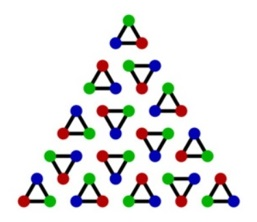To glean further information about the structure of the partition, focus on the red and blue points, and the unit segments not contained by the side of any triangle in the partition.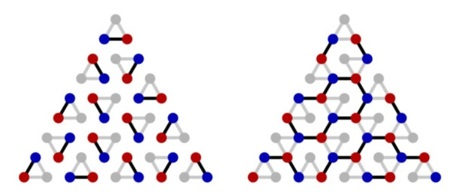One can conjecture that they will form a collection of paths. If so, we could fuse these paths together by extending the grid by $\displaystyle 1$ point on each side. Hence, we will rather consider this extension: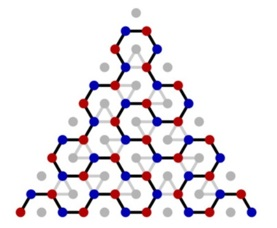Lemma. Consider the graph $\displaystyle G$ whose vertices are red or blue points in the extended coloring and whose edges are the unit segments connecting red and blue points not contained by any side in the partition. Then this graph is its own Hamiltonian path.

Proof. $\displaystyle G$ has two nodes with degree $\displaystyle 1$, namely the blue vertex $\displaystyle v_b$ and the red vertex $\displaystyle v_r$ of the extended triangle; all other nodes have degree $\displaystyle 2$. Every such graph can be decomposed into a path and a collection of cycles. The path will connect $\displaystyle v_b$ and $\displaystyle v_r$; we claim that no cycle can occur.

Suppose that there exists some cycle in $\displaystyle G$, and consider the region $\displaystyle \mathcal{R}$ enclosed by it. Note that $\displaystyle \mathcal{R}$ is the union of the hexagons of side-length $\displaystyle 1$ centered at each green point in $\displaystyle \mathcal{R}$. Each green point is among the original $\displaystyle 1+2+\dots+(n+1)$ points, and so is the vertex of some triangle from the partition. Hence, the number of triples in $\displaystyle \mathcal{R}$ is equal to the number of hexagons in $\displaystyle \mathcal{R}$.

However, in this case, if we start from a hexagon in $\displaystyle \mathcal{R}$, since it contains a green point, one of its sides is the red-blue side of the corresponding triple. Take the other hexagon neighboring this side; the green point at its center defines a different red-blue side and a further hexagon. Continuing this way, we must eventually enter a cycle. When we connect the neighboring green vertices of the cycle, we get a curve whose interior contains at least one red or blue point, and that point must be connected to some neighboring blue or red point. So there is an edge of $\displaystyle G$ inside $\displaystyle \mathcal{R}$, and we know this edge must be part of a cycle.

We thus reach a contradiction if we begin with the cycle enclosing minimal area. $\displaystyle \blacksquare$

Our final crucial observation is the following. Begin from a broken line connecting $\displaystyle v_b$ and $\displaystyle v_r$ with length $\displaystyle 4k$ for $\displaystyle n=3k-1$ and length $\displaystyle 4k+2$ for $\displaystyle n=3k+1$: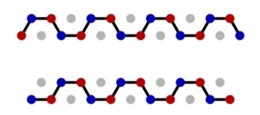Of the edges on the broken line, we may repeatedly replace an edge which is the side of a triple by the $\displaystyle 5$ further edges of the unit-side hexagon centered at the green point of the triple, until we reach the complete Hamiltonian path. This is because every resulting path connects $\displaystyle v_b$ and $\displaystyle v_r$, and by the Lemma, this path has a red-blue side of a triple on it unless it is the Hamiltonian path of $\displaystyle G$. In this case, the green point of this triple must be the center of a hexagon we haven't yet adjoined, so we can continue the process.

If $\displaystyle n=3k-1$, the path thus has length of the form $\displaystyle 4x$, so

$\displaystyle 4\text{ divides }\frac23\cdot \frac{(n+4)(n+5)}{2},$

i.e. $\displaystyle \frac{(n+4)(n+5)}{2}$ is even, so $\displaystyle n$ is $\displaystyle -1$ or $\displaystyle 0$ mod $\displaystyle 4$, and thus $\displaystyle -1$ or $\displaystyle 8$ mod $\displaystyle 12$.

If $\displaystyle n=3k+1$, the path must have length of the form $\displaystyle 4x+2$, so $\displaystyle \frac{(n+4)(n+5)}{2}$ is now odd, so $\displaystyle n$ is $\displaystyle 1$ or $\displaystyle 2$ mod $\displaystyle 4$, and thus $\displaystyle 1$ or $\displaystyle 10$ mod $\displaystyle 12$.

This completes the proof.

### Statistics:

 15 students sent a solution. 5 points: Schrettner Jakab. 2 points: 3 students. 1 point: 4 students. 0 point: 7 students.

Problems in Mathematics of KöMaL, October 2017